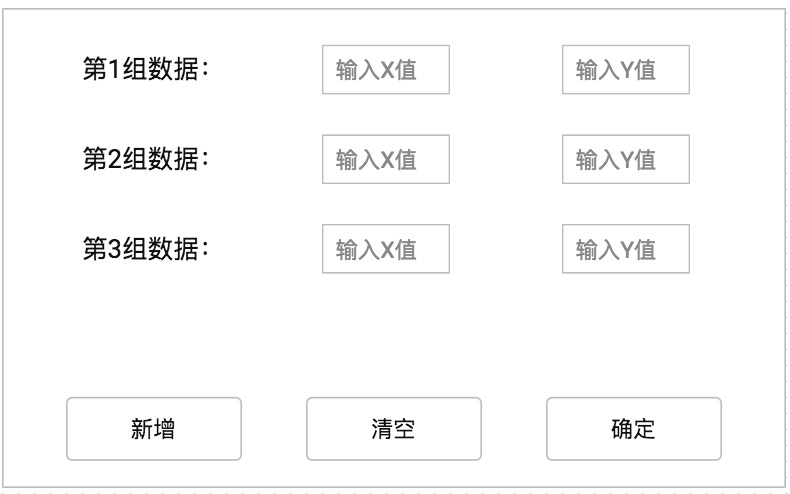###### fanshinee

2018-07-03 01:45 阅读 3.8k

# 如何在选择一个选项后，有选择的弹出输入框

5

from tkinter import *
root = Tk()
root.title("元素周期表")
def cal():
master = Tk()
v1=StringVar()
v2=StringVar()
v3=StringVar()
frame = Frame(master)
frame.pack(padx=10,pady=10)
Entry(frame,width=10,textvariable=v1).grid(row = 0, column = 1)
Entry(frame,width=10,textvariable=v2).grid(row = 1, column = 1)
Entry(frame,width=10,textvariable=v3).grid(row = 2, column = 1)
Button(frame,text="数1").grid(row=0,column=0)
Button(frame,text="数2").grid(row=1,column=0)
Button(frame,text="数3").grid(row=2,column=0)

Button(root,text="选择",command=cal).grid(row=2,column=1)
Button(root,text="输入一个数",command=cal).grid(row=2,column=1)
Button(root,text="输入另一个数",command=cal).grid(row=2,column=2)

mainloop()

• 点赞
• 写回答
• 关注问题
• 收藏
• 复制链接分享

#### 3条回答默认 最新

• 已采纳
• `````` from tkinter import *

root = Tk()
root.title("元素周期表")

def cal(type):
master = Tk()
v1 = StringVar()
v2 = StringVar()
v3 = StringVar()
frame = Frame(master)
frame.pack(padx=10, pady=10)

Entry(frame, width=10, textvariable=v1).grid(row=0, column=1)
Button(frame, text="数1").grid(row=0, column=0)

if (type == 1): return;
Entry(frame, width=10, textvariable=v2).grid(row=1, column=1)
Button(frame, text="数2").grid(row=1, column=0)

if (type == 2): return;
Entry(frame, width=10, textvariable=v3).grid(row=2, column=1)
Button(frame, text="数3").grid(row=2, column=0)

Button(root, text="选择", command=cal).grid(row=2, column=1)
Button(root, text="输入一个数", command=lambda :cal(1)).grid(row=2, column=1)
Button(root, text="输入另一个数", command=lambda :cal(2)).grid(row=2, column=2)

mainloop()
``````
点赞 评论 复制链接分享
•希望完成的是点击增加，能够实现增加一行（第四组数据+两个输入框），这些框可以自动向下排列；另外，这些框中数据可以运算，如第一行的xy相乘然后加上第二行的xy乘积，一直到最后一行；
点击清空，可以去除一行
点击确定则进行输出结果，放在最后一行

点赞 评论 复制链接分享